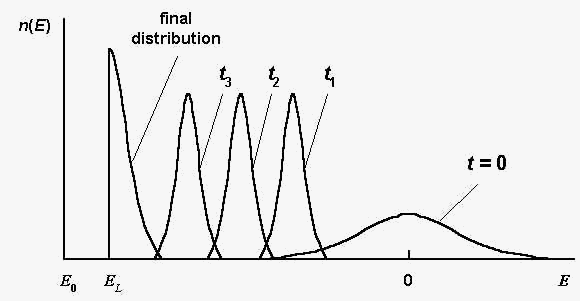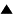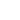# Spin-glass model of evolution

The model of evolution, discussed in Quasispecies, implies a strong assumption: the selective value is determined by the Hamming distance between the particular and unique master sequences. Only one maximum of the selective value exists. Using the physical spin-glass concept, we can construct a similar model for a very large number of the local maxima of a selective value.

D. Sherrington and S. Kirkpatrick proposed a simple spin-glass model to interpret the physical properties of the systems, consisting of randomly interacting spins . This well-known model can be described shortly as follows:
 ; 1) There is a system S of spins Si , i = 1,..., N (the number of spins N is supposed to be large, N >> 1). Spins take the values: Si = 1, -1 . ; 2) The exchange interactions between spins are random. The energy of the spin system is defined as:
 E (S) = - Si
 where Jij are the exchange interactions matrix elements. Jij are normally distributed random values:
 Probability_density{Jij } = (2p)-1/2 (N-1)1/2 exp [- Jij2 (N-1)2-1] . (2)

From (1), (2) one can obtain, that the mean spin-glass energy is zero (<E > = 0) , and the mean square root value of the energy variation at one-spin reversal is equal to 2:
 MSQR {dE (Si --> - Si) } = 2 . (3)

The model (1), (2) was intensively investigated. For further consideration the following spin-glass features are essential :

- the number of local energy minima M is very large: M ~ exp(aN) , a = 0,2 ; (a local energy minimum is defined as a state SL , at which any one-spin reversal increases the energy E );

- the global energy minimum E0 equals approximately: E0 = - 0,8 N .

Let's construct the spin-glass model of evolution . We suppose, that an informational sequence (a genome of a model "organism") can be represented by a spin-glass system vector S . The evolved population is a set {Sk} of n sequences, k = 1,..., n. The selective values of model "organisms" Sk are defined as:
 f(Sk) = exp[- b E(Sk)] , (4)

where b is the selection intensity parameter.

The definition (4) implies that the model genome Sk consists of different elements Ski , which pairwise interact in accordance with the random interaction matrix Jij . In order to maximize the "organism" selective value (that is to minimize the energy E(S)), it is necessary to find a such combination of elements Si , that provides maximally cooperative interactions for given matrix Jij .

As in Quasispecies , we suppose, that 1) the evolution process consists of consequent generations, 2) new generations are obtained by selection (in accordance with selective values (4)) and mutations (sign reversals of sequence symbols, Ski --> - Ski , with the probability P for any symbol) of sequences Sk . The initial population is supposed to be random.

The described spin-glass model of evolution was analyzed by means of computer simulations and rough estimations . The main evolution features are illustrated by Fig.1. Here n(E) is the number of sequences S , such that E(S) = E in a considered population; t is the generation number.Fig. 1. The sequence distribution n(E) at different generations t ; t3 > t2 > t1 ; E0 and EL are global and local energy minima, respectively; the global energy minimum E0 equals approximately: E0 = - 0,8 N. Schematically, according to the computer simulations .

The spin-glass-type evolution is analogous to the Hamming distance case (see Quasispecies, Estimation of the evolution rate). But unlike the Hamming-distance model, the evolution converges to one of the local energy minima EL , which can be different for different evolution realizations.

Because one mutation gives the energy change dE ~ 2 (see (3)), and the typical time for one mutation per sequence dt is of the order (PN)-1, the total number of evolution generations T (at sufficiently large selection intensity b) can be estimated by the value T ~ (|E0|/dE)xdt ~ (0.8N /2)x(PN)-1. This value is close to the estimation of value T in the Hamming-distance case. So, the estimations of the evolution rate are roughly the same for both models, and we can use formulas (1)-(3) in Quasispecies to characterize the spin-glass-type evolution as well.

Analogously to the Hamming-distance case, we can consider the sequential method of energy minimization, that is the consequent changes of symbols (Si --> - Si) of one sequence and fixation only successful reversals. The sequential search needs smaller participant number than the evolution search. Nevertheless, the evolution search provides in average a more deep local energy minimum EL , because different valleys in energy landscape are looked through in evolution process simultaneously with descending to energy minima.

Thus, in the spin-glass case, the evolutionary search has a certain advantage with respect to the sequential search: it provides in average the greater selective value.

Conclusion. The spin-glass model of evolution refers to the "organisms", which have many randomly interacting genome elements. Evolution can be considered as a search of such genome elements, which are able to cooperate in the most successful manner.

References:

1. D.Sherrington , S.Kirkpatrick. // Physical Review Letters. 1975. V.35. N.26. P.1792. S.Kirkpatrick, D.Sherrington. // Physical Review B. 1978. V.17. N.11. P.4384.

2.V.G.Red'ko. Biofizika. 1990. Vol. 35. N.5. P. 831 (In Russian).

 HomeMetasystem Transition TheoryEvolutionary TheoryMathematical Modeling of EvolutionModels of molecular-genetic systems originQuasispecies Up Prev.Next Down